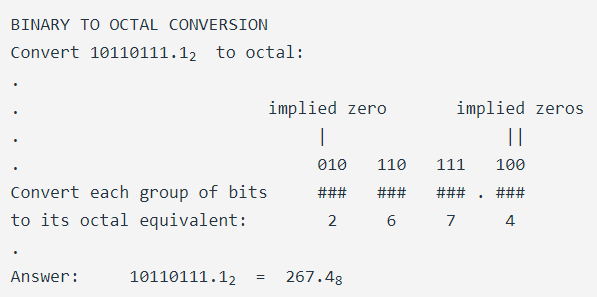## What is octal?

A place-weighted system with a base of eight.

## Chapter 1 - Numeration Systems

Because binary numeration requires so many bits to represent relatively small numbers compared to the economy of the decimal system, analyzing the numerical states inside of digital electronic circuitry can be a tedious task.

Computer programmers who design sequences of number codes instructing a computer what to do would have a very difficult task if they were forced to work with nothing but long strings of 1’s and 0’s, the “native language” of any digital circuit.

To make it easier for human engineers, technicians, and programmers to “speak” this language of the digital world, other systems of place-weighted numeration have been made which are very easy to convert to and from binary.

One of those numeration systems is called octal, because it is a place-weighted system with a base of eight. Valid ciphers include the symbols 0, 1, 2, 3, 4, 5, 6, and 7. Each place weight differs from the one next to it by a factor of eight.

Another system is called hexadecimal, because it is a place-weighted system with a base of sixteen.

Valid ciphers include the normal decimal symbols 0, 1, 2, 3, 4, 5, 6, 7, 8, and 9, plus six alphabetical characters A, B, C, D, E, and F, to make a total of sixteen.

As you might have guessed already, each place weight differs from the one before it by a factor of sixteen.

Let’s count again from zero to twenty using decimal, binary, octal, and hexadecimal to contrast these systems of numeration:

Number       Decimal      Binary        Octal      Hexadecimal
------       -------      -------       -----      -----------
Zero            0           0             0             0
One             1           1             1             1
Two             2           10            2             2
Three           3           11            3             3
Four            4           100           4             4
Five            5           101           5             5
Six             6           110           6             6
Seven           7           111           7             7
Eight           8           1000          10            8
Nine            9           1001          11            9
Ten             10          1010          12            A
Eleven          11          1011          13            B
Twelve          12          1100          14            C
Thirteen        13          1101          15            D
Fourteen        14          1110          16            E
Fifteen         15          1111          17            F
Sixteen         16          10000         20            10
Seventeen       17          10001         21            11
Eighteen        18          10010         22            12
Nineteen        19          10011         23            13
Twenty          20          10100         24            14


Octal and hexadecimal numeration systems would be pointless if not for their ability to be easily converted to and from binary notation. Their primary purpose in being is to serve as a “shorthand” method of denoting a number represented electronically in binary form.

Because the bases of octal (eight) and hexadecimal (sixteen) are even multiples of binary’s base (two), binary bits can be grouped together and directly converted to or from their respective octal or hexadecimal digits. With octal, the binary bits are grouped in three’s (because 23 = 8), and with hexadecimal, the binary bits are grouped in four’s (because 24 = 16):

### Binary to Octal Conversion

BINARY TO OCTAL CONVERSION
Convert 10110111.12  to octal:
.
.                         implied zero       implied zeros
.                              |                  ||
.                              010   110   111   100
Convert each group of bits     ###   ###   ### . ###
to its octal equivalent:        2     6     7     4
.


We had to group the bits in three’s, from the binary point left, and from the binary point right, adding (implied) zeros as necessary to make complete 3-bit groups. Each octal digit was translated from the 3-bit binary groups.

Binary-to-Hexadecimal conversion is much the same:

BINARY TO HEXADECIMAL CONVERSION
.
.                                        implied zeros
.                                             |||
.                              1011   0111   1000
Convert each group of bits     ----   ---- . ----
to its hexadecimal equivalent:   B      7      8
.


Here we had to group the bits in four’s, from the binary point left, and from the binary point right, adding (implied) zeros as necessary to make complete 4-bit groups:

Likewise, the conversion from either octal or hexadecimal to binary is done by taking each octal or hexadecimal digit and converting it to its equivalent binary (3 or 4 bit) group, then putting all the binary bit groups together.

Incidentally, hexadecimal notation is more popular, because binary bit groupings in digital equipment are commonly multiples of eight (8, 16, 32, 64, and 128 bit), which are also multiples of 4. Octal, being based on binary bit groups of 3, doesn’t work out evenly with those common bit group sizings.

RELATED WORKSHEETS:

• Share
Published under the terms and conditions of the Design Science License